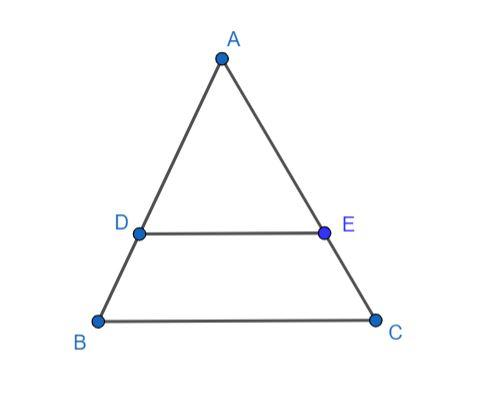QuestionAnswers

# In a triangle ABC, DE is parallel to BC. If $\dfrac{{AD}}{{DB}} = \dfrac{2}{3}$ and AC = 18 cm, then find AE.Hint: Draw triangle ABC and DE parallel to BC. Prove that the two triangles are similar and then use the properties of similar triangles to find the relation between AE and AC and then find AE.In the diagram, ABC is a triangle and the line segment DE is parallel to BC.
Let us check triangle ABC and triangle ADE for similarity.
We know that corresponding angles on two parallel lines are equal. Hence, angle ADE is equal to angle ABC and angle AED is equal to angle ACB.
$\angle ADE = \angle ABC..............(1)$
$\angle AED = \angle ACB..............(2)$
Angle A is common between both the triangles. Hence, we have:
$\angle EAD = \angle BAC..............(3)$
From equation (1), equation (2) and equation (3), the triangles ABC and ADE are similar by Angle-Angle-Angle (AAA) criterion. Hence, we have:
$\Delta ABC \sim \Delta ADE$
We know that the properties of similar triangles states that the ratio of corresponding sides of similar triangles are equal.
For triangle ABC and triangle ADE, we have AB and AD, AC and AE, BC and DE as corresponding sides. Hence, the ratio of AB by AD is equal to the ratio of AC by AE is equal to the ratio of BC by DE.
Hence, we have as follows:
$\dfrac{{AB}}{{AD}} = \dfrac{{AC}}{{AE}} = \dfrac{{BC}}{{DE}}$
Now, considering the first two equal terms we have:
$\dfrac{{AB}}{{AD}} = \dfrac{{AC}}{{AE}}$
We know that AB = AD + DB, then, we have:
$\dfrac{{AD + DB}}{{AD}} = \dfrac{{AC}}{{AE}}$
Simplifying, we have:
$1 + \dfrac{{DB}}{{AD}} = \dfrac{{AC}}{{AE}}$
It is given that $\dfrac{{AD}}{{DB}} = \dfrac{2}{3}$ and AC = 18 cm. Hence, we have:
$1 + \dfrac{3}{2} = \dfrac{{18}}{{AE}}$
Simplifying, we have:
$\dfrac{5}{2} = \dfrac{{18}}{{AE}}$
Solving for AE, we have:
$AE = \dfrac{2}{5} \times 18$
$AE = 7.2cm$
Hence, the value of AE is 7.2 cm.

Note: You can also prove the similarity by other criteria. The ratio of AD to DB is only given, hence, you can either express AB as AD + DB or you can solve to find the ratio of AB to AD.
View Notes
De Morgan's First LawCapacitors in ParallelWhere There is a Will There is a Way EssayResistors in Series and ParallelCBSE Class 10 Maths Chapter 6 - Triangles FormulaWave Nature of Matter and De Broglie’s EquationCBSE Class 10 Maths Chapter 8 - Introduction to Trigonometry FormulaIndian Olympiad Qualifier in Mathematics- IOQM (Similar to PRMO of Last Year)CBSE Class 10 Maths Chapter 13 - Surface Areas and Volumes FormulaThe Difference Between an Animal that is A Regulator and One that is A ConformerImportant Questions for CBSE Class 10 Maths Chapter 12 - Areas Related to CirclesImportant Questions for CBSE Class 10 Maths Chapter 8 - Introduction to TrigonometryImportant Questions for CBSE Class 10 Maths Chapter 6 - TrianglesImportant Questions for CBSE Class 8 English Honeydew Chapter 7 - A Visit to CambridgeImportant Questions for CBSE Class 9 Maths Chapter 9 - Areas of Parallelograms and TrianglesImportant Questions for CBSE Class 10 Maths Chapter 3 - Pair of Linear Equations in Two VariablesImportant Questions for CBSE Class 10 Maths Chapter 13 - Surface Areas and VolumesImportant Questions for CBSE Class 10 Maths Chapter 10 - CirclesImportant Questions for CBSE Class 6 English A Pact with The Sun Chapter 10 - A Strange Wrestling MatchImportant Questions for CBSE Class 11 Physics Chapter 4 - Motion in a PlaneCBSE Class 10 Hindi A Question Paper 2020Hindi A Class 10 CBSE Question Paper 2009Hindi A Class 10 CBSE Question Paper 2015Hindi A Class 10 CBSE Question Paper 2016Hindi A Class 10 CBSE Question Paper 2012Hindi A Class 10 CBSE Question Paper 2010Hindi A Class 10 CBSE Question Paper 2007Hindi A Class 10 CBSE Question Paper 2013Hindi A Class 10 CBSE Question Paper 2008Hindi A Class 10 CBSE Question Paper 2014RS Aggarwal Class 9 Solutions Chapter-9 Congruence of Triangles and Inequalities in a TriangleNCERT Solutions for Class 10 Maths Chapter 12 Areas Related to CirclesRD Sharma Solutions for Class 10 Maths Chapter 15 - Areas Related to CirclesNCERT Solutions for Class 10 Maths Chapter 12 Areas Related to Circles in HindiNCERT Solutions for Class 9 Maths Chapter 9 Areas of Parallelograms and TrianglesRD Sharma Solutions for Class 9 Maths Chapter 15 - Areas of Parallelograms and TrianglesNCERT Solutions for Class 10 Maths Chapter 6 TrianglesNCERT Solutions for Class 10 Maths Chapter 13 Surface Areas and VolumesNCERT Solutions for Class 10 Maths Chapter 8 Introduction to Trigonometry in HindiRS Aggarwal Class 10 Solutions - Triangles